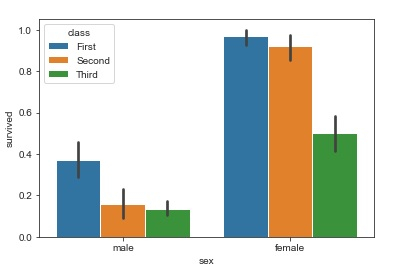# How can bar plot be used in Seaborn library in Python?

Seaborn is a library that helps in visualizing data. It comes with customized themes and a high level interface.

In previous plots, we plotted the entire dataset on the graph. With the help of bar plots, we can understand the central tendency of the distribution of data.

The barplot function establishes the relationship between a categorical variable and a continuous variable. Data is represented in the form of rectangular bars where the length of the bar indicates the proportion of data in that specific category.

Let us understand bar plots with the help of ‘titanic’ dataset −

## Example

import pandas as pd
import seaborn as sb
from matplotlib import pyplot as plt
sb.barplot(x = "sex", y = "survived", hue = "class", data = my_df)
plt.show()

## Output## Explanation

• The required packages are imported.
• The input data is ‘titanic’ which is loaded from the seaborn library.
• This data is stored in a dataframe.
• This data is visualized using the ‘barplot’ function.
• Here, the dataframe is supplied as parameter.
• Also, the x and y values are specified.
• This data is displayed on the console.

Updated on: 11-Dec-2020

64 Views